Cellular AutomataNext: Dynamic Model Up: No Title Previous: Fluid Dynamics

# Cellular Automata

Cellular Automata have the advantage of modeling the traffic flow on the microscopic scale of individual vehicles and allow the study of large systems due to a simple type of dynamics. A one-dimensional model with periodic boundary conditions representing a single lane freeway was introduced by Nagel el al. [8, 9] and later slightly modified in . The sites of the array of length L can be occupied by a vehicle with velocity between zero and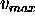or be empty. The system is updated in each time step performing the following steps:

1. Free travel: A car traveling withand sufficient free space ahead keeps this velocity and the two following steps are skipped,
2. Acceleration: If the distance to the next car is big enough and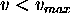, v is increased by 1 with a probability of,
3. Slowing down: If there is another car j sites ahead the velocity is reduced to j-1. With probability of, it overacts and slows down even further to j-2,
4. Movement: Each vehicle advances v sites.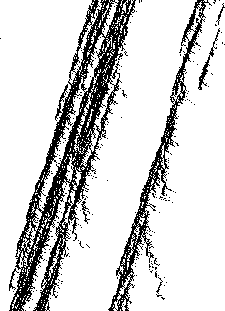Figure 4: Space-time plot of a traffic jam in the cellular automata model by Nagel el al. . The horizontal direction is space and the vertical time. Only the position of cars in jams are shown.

This model has been studied intensively using parallel computers, in particular, the lifetime of emerging traffic jams such as shown in Fig. 4. It was shown that the probability distribution as a function of lifetime follows a power law,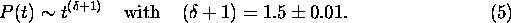This exponent is very close to, which is the first return-time exponent for a one-dimensional random walk. This hypothesis was pursued by studying the probability distribution P(n,t) of a jam of size n at time t analytically. The time-dependence of the this distribution is given by,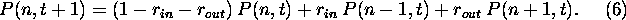This equation describes the growing and shrinking of a jam, where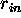and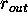are density dependent parameters for the rate of cars driving in and out of the jam. From this equation, the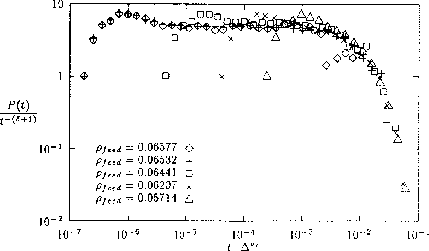Figure 5:   Data collapse for the lifetime distribution of jams in the cellular automata model by Nagel el al. .

analogy to a one-dimensional random walk becomes clear. A jam starts at size n=1 at particular time. It grows, then shrinks and finally disappears if n is equal to one at a later time. This is the reason why the life time of a jam can be related to first return-time of one-dimensional random walk. Going to the continuum limit in Eq. 6, it can be shown that P follows a scaling function,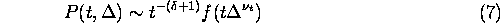near the critical point with critical density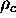.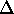is defined as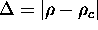. In the case of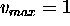, it follows exactly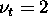and. For the numerical results for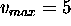, a data collapse was performed using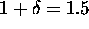(Fig. 5). It was found,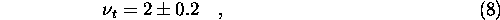which agrees with prediction from the random walk model.Next: Dynamic Model Up: No Title Previous: Fluid Dynamics

Burkhard Militzer
Sat May 9 11:34:31 CDT 1998